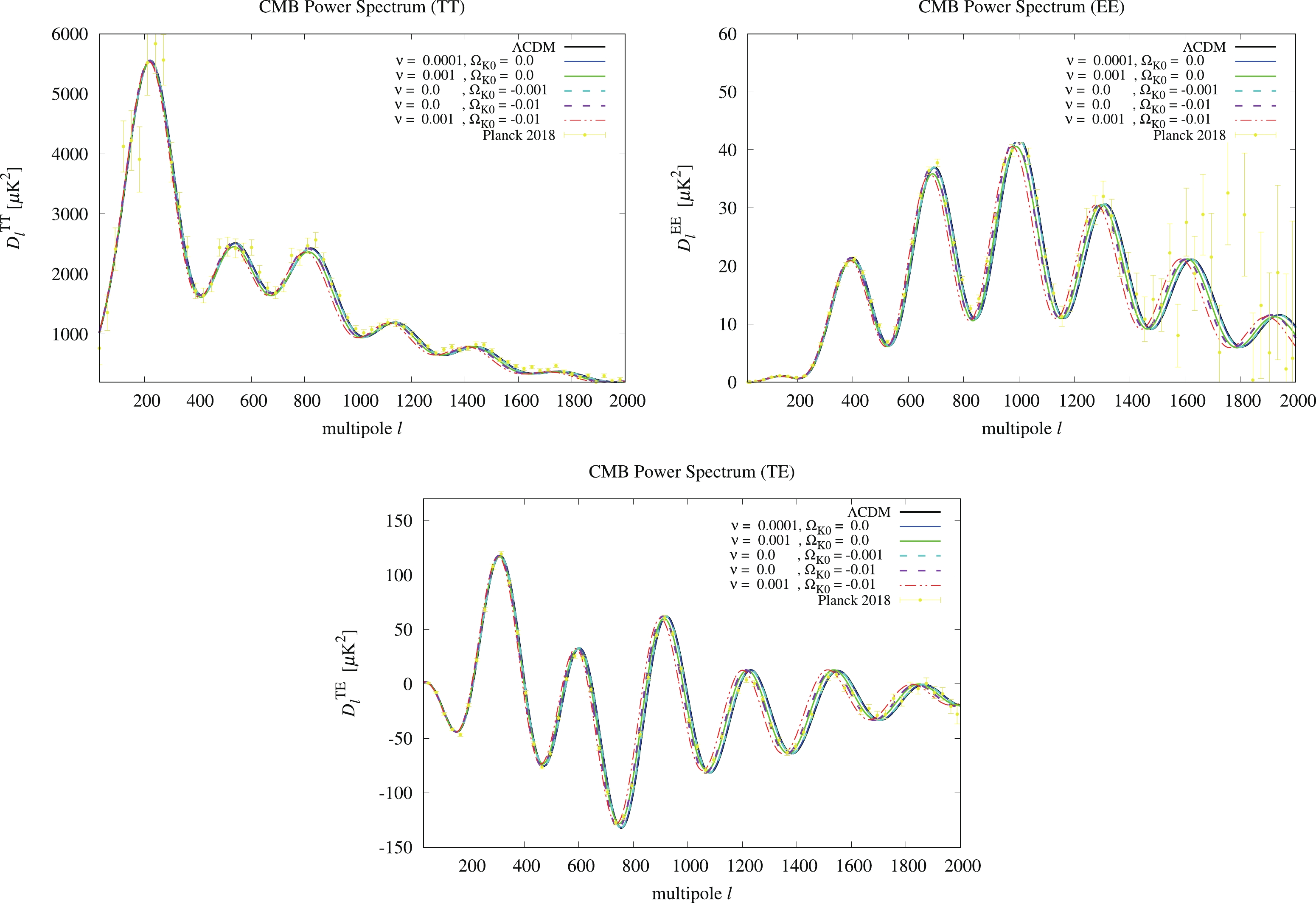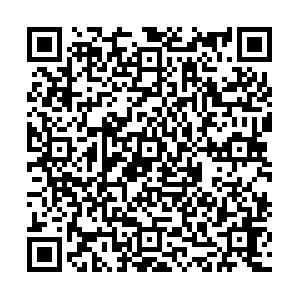# Running vacuum model in a non-flat universe

• We investigate observational constraints on the running vacuum model (RVM) of $\Lambda=3\nu (H^{2}+K/a^2)+c_0$in a spatially curved universe, where $\nu$is the model parameter, $K$corresponds to the spatial curvature constant, $a$represents the scalar factor, and $c_{0}$is a constant defined by the boundary conditions. We study the CMB power spectra with several sets of $\nu$and $K$in the RVM. By fitting the cosmological data, we find that the best fitted $\chi^2$value for RVM is slightly smaller than that of $\Lambda$CDM in the non-flat universe, along with the constraints of $\nu\leqslant O(10^{-4})$(68% C.L.) and $|\Omega_K=-K/(aH)^2|\leqslant O(10^{-2})$(95% C.L.). In particular, our results favor the open universe in both $\Lambda$CDM and RVM. In addition, we show that the cosmological constraints of $\Sigma m_{\nu}=0.256^{+0.224}_{-0.234}$(RVM) and $\Sigma m_{\nu}=0.257^{+0.219}_{-0.234}$($\Lambda$CDM) at 95% C.L. for the neutrino mass sum are relaxed in both models in the spatially curved universe.
••Get Citation
Chao-Qiang Geng, Yan-Ting Hsu, Lu Yin and Kaituo Zhang. Running vacuum model in non-flat universe[J]. Chinese Physics C. doi: 10.1088/1674-1137/abab86
Chao-Qiang Geng, Yan-Ting Hsu, Lu Yin and Kaituo Zhang. Running vacuum model in non-flat universe[J]. Chinese Physics C.Milestone
Received: 2020-03-19
Revised: 2020-06-07
Article Metric

Article Views(237)
PDF Downloads(18)
Cited by(0)
Policy on re-use
To reuse of subscription content published by CPC, the users need to request permission from CPC, unless the content was published under an Open Access license which automatically permits that type of reuse.
###### 通讯作者: 陈斌, bchen63@163.com
• 1.

沈阳化工大学材料科学与工程学院 沈阳 110142

Title:
Email:

## Running vacuum model in a non-flat universe

###### Corresponding author: Kaituo Zhang, ktzhang@ahnu.edu.cn, Corresponding author
• 1. School of Fundamental Physics and Mathematical Sciences Hangzhou Institute for Advanced Study, UCAS, Hangzhou 310024
• 2. International Centre for Theoretical Physics Asia-Pacific, Beijing/Hangzhou
• 3. Department of Physics, National Tsing Hua University, Hsinchu, 300
• 4. National Center for Theoretical Sciences, Hsinchu, 300
• 5. Department of Physics, Anhui Normal University, Wuhu, Anhui 241000
• 6. Collaborative Innovation Center of Light Manipulations and Applications, Shandong Normal University, Jinan 250358

Abstract: We investigate observational constraints on the running vacuum model (RVM) of $\Lambda=3\nu (H^{2}+K/a^2)+c_0$in a spatially curved universe, where $\nu$is the model parameter, $K$corresponds to the spatial curvature constant, $a$represents the scalar factor, and $c_{0}$is a constant defined by the boundary conditions. We study the CMB power spectra with several sets of $\nu$and $K$in the RVM. By fitting the cosmological data, we find that the best fitted $\chi^2$value for RVM is slightly smaller than that of $\Lambda$CDM in the non-flat universe, along with the constraints of $\nu\leqslant O(10^{-4})$(68% C.L.) and $|\Omega_K=-K/(aH)^2|\leqslant O(10^{-2})$(95% C.L.). In particular, our results favor the open universe in both $\Lambda$CDM and RVM. In addition, we show that the cosmological constraints of $\Sigma m_{\nu}=0.256^{+0.224}_{-0.234}$(RVM) and $\Sigma m_{\nu}=0.257^{+0.219}_{-0.234}$($\Lambda$CDM) at 95% C.L. for the neutrino mass sum are relaxed in both models in the spatially curved universe.

### HTML2.   Evolution of RVM in a curved universe3.   Numerical calculations
Reference (85)
PDF查看关注分享

Top

### 目录/DownLoad:  Full-Size Img  PowerPoint